0
196

# Data Interpretation Graphs Questions for MAH-CET

Question 1: Study the following graph and answer the question that follows.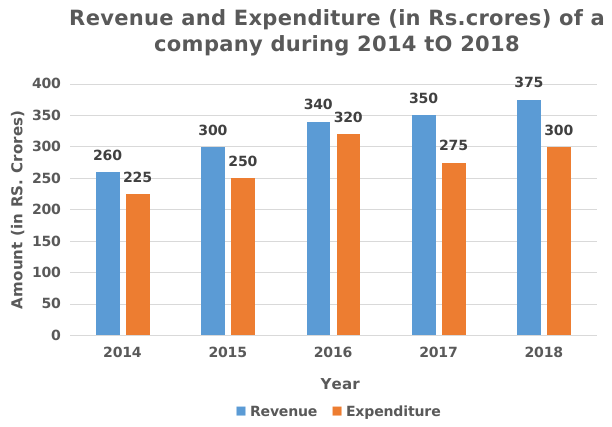What is the total ratio of the revenue of the company in 2016 and 2018 to that of the total expenditure in 2015 and 2017 respectively?

a) 143:105

b) 130:103

c) 115:114

d) 105:143

Solution:

Total revenue of the company in 2016 and 2018 = 340 + 375 = 715

Total expenditure of the company in 2015 and 2017 = 250 + 275 = 525

Required Ratio = 715 : 525

i.e; 143 : 105

Hence, option A is correct.

Question 2: Study the following graph and answer question that follows.The expenditure of the company in 2016 is what percentage more then the average revenue (per year) in 2014, 2015 and 2018 (correct to the nearest integer)?

a) 3%

b) 5%

c) 6%

d) 4%

Solution:

Expenditure of the company in 2016 = 320

Average revenue in 2014,2015 and 2018

$\therefore\frac{\left(revenue\ in\ 2014+revenue\ in\ 2015+revenue\ in\ 2018\right)}{3}$

$\therefore\frac{\left(260+300+375\right)}{3}$

= 311.67

Required percentage = $\frac{\left(320-311.67\right)}{311.67}$

$=2.67\simeq\ 3\%$

Hence, option A is correct

Question 3: The bar graph given below shows the sales of Newspapers (in lakh number) from six branches of a Media Publication Company during two consecutive years 2017 and 2018.
(Note: The data shown below is only for mathematical exercise. They do not represent the actual figures).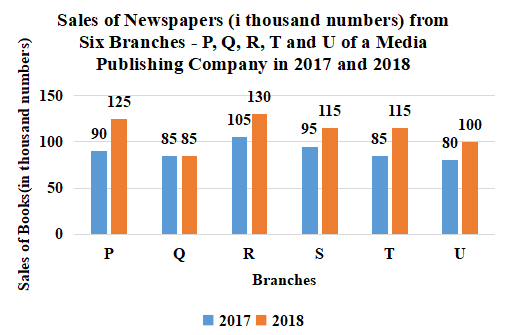Total Sales of U for both the years is what percent (correct to one place of decimal) of the combined Sales of the branches Q and R for 2017 and 2018?

a) 48.6%

b) 67.1%

c) 44.4%

d) 41.0%

Solution:

Total Sales of U for both the years = 80 + 100

= 180

Total sales of Q for 2017 and 2018 = 85 + 85

= 170

Total sales of R for 2017 and 2018 = 105 + 130

= 235

The combined Sales of the branches Q and R for 2017 and 2018 = 170 + 235

= 405

Required percentage = $\frac{180}{405}\times$100

= 44.4%

Hence, the correct answer is Option C

Question 4: The pie graph shows the distribution of employees working in five departments A, B, C, D and E of a company.
Total number of employees = 9000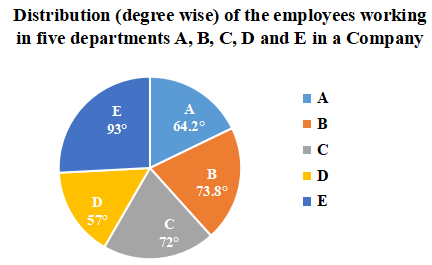If the number of employees working in department A is x and the total number of employees working in departments C and E is y, then the value of y – 2x is:

a) 725

b) 850

c) 1000

d) 915

Solution:

Number of employees working in department A = x = $\frac{64.2^{\circ\ }}{360^{\circ\ }}\times9000$ = 1605

x = 1605

Number of employees working in department C = $\frac{72^{\circ\ }}{360^{\circ\ }}\times9000$ = 1800

Number of employees working in department E = $\frac{93^{\circ\ }}{360^{\circ\ }}\times9000$ = 2325

The total number of employees working in departments C and E = y = 1800 + 2325 = 4125

y = 4125

y – 2x = 4125 – 2(1605) = 4125 – 3210 = 915

Hence, the correct answer is Option D

Question 5: Bar graph shows the number of males and females in five organizations A, B, C, D and E.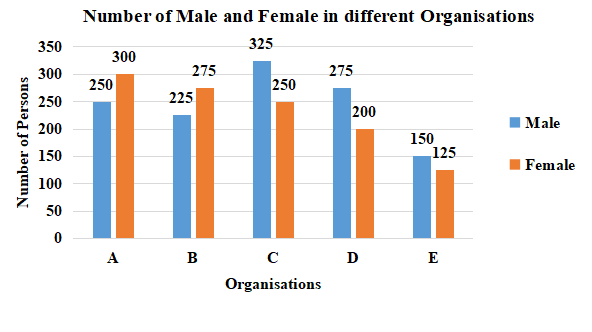What is the ratio of number of males working in organizations C, D and E taken together to that of females working in organizations A, B and C taken together?

a) 10:11

b) 49:46

c) 11:10

d) 46:49

Solution:

Number of males working in organizations C, D and E taken together = 325 + 275 + 150 = 750

Number of females working in organizations A, B and C taken together = 300 + 275 + 250 = 825

Required ratio = 750 : 825

= 10 : 11

Hence, the correct answer is Option A

Take Free MAH-CET mock tests here

Question 6: The following bar graph shows the amount(in Lakh Rs.) invested by a Company in purchasing raw material over the years and the values (in Lakh Rs.) of finished goods sold by the Company over the years.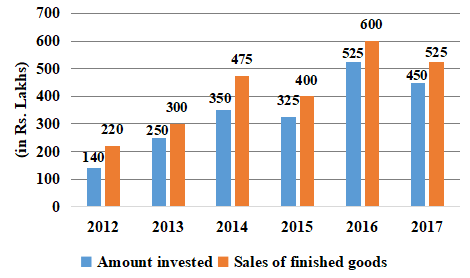The ratio of total amount invested for purchasing raw material from 2013 to 2015 to the total sales of finished goods in 2014, 2016 and 2017 is:

a) 27 : 56

b) 56 : 27

c) 64 : 37

d) 37 : 64

Solution:

Total amount invested for purchasing raw material from 2013 to 2015 = 250 + 350 + 325 = 925

The total sales of finished goods in 2014, 2016 and 2017 = 475 + 600 + 525 = 1600

Required ratio = 925 : 1600

= 37 : 64

Hence, the correct answer is Option D

Question 7: The given histogram represents the marks obtained by 128 students. Read the graph and answer the question that follows.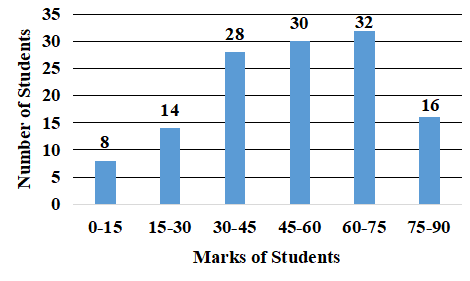What percent of students got marks less than 60?

a) 67.5%

b) 72.5%

c) 75%

d) 62.5%

Solution:

From the Histogram,

Number of students who got less than 60 marks = 8 + 14 + 28 + 30

= 80

Required percentage = $\frac{80}{128}\times$100

= 62.5%

Hence, the correct answer is Option D

Question 8: Bar graph shows the number of males and females in five organizations A, B, C, D and E.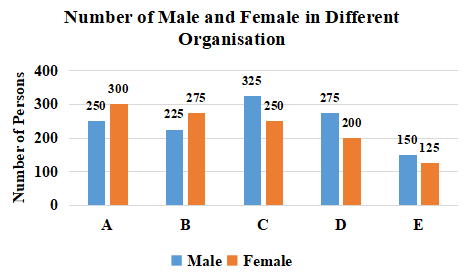For which organisation, difference between the number of males and the average number of females of all the organisations is minimum?

a) D

b) C

c) A

d) B

Solution:

The average number of females in all the organisations = $\frac{300+275+250+200+125}{5}$ = $\frac{1150}{5}$ = 230

For Organisation A, difference between the number of males and the average number of females of all the organisations = 250 – 230 = 20

For Organisation B, difference between the number of males and the average number of females of all the organisations = 230 – 225 = 5

For Organisation C, difference between the number of males and the average number of females of all the organisations = 325 – 230 = 95

For Organisation D, difference between the number of males and the average number of females of all the organisations = 275 – 230 = 45

$\therefore$  For Organisation B, difference between the number of males and the average number of females of all the organisations is minimum.

Hence, the correct answer is Option D

Question 9: Study the following graph and answer the given question.
The graph shows the time (in minutes) taken by the pipes (A, B), (C, D), (E, F), (G, H) and (P, Q) to fill a tank.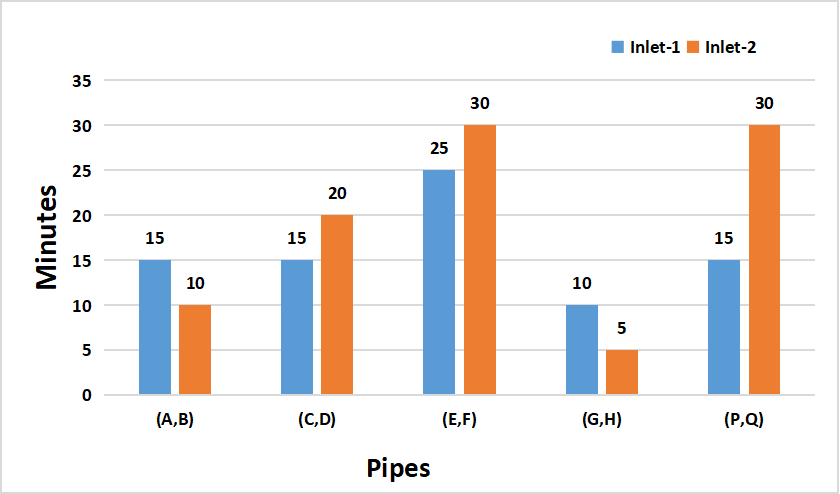In how many minutes is an empty tank filled completely if pipe D fills it for half the time and then for the other half time, pipes C and D fill it together?

a) 18 minutes

b) 10 minutes

c) 15 minutes

d) 12 minutes

Solution:

From the graph,

Time taken by the pipe C to fill the tank = 15 minutes

Time taken by the pipe D to fill the tank = 20 minutes

Let the Volume of the tank = 60 units(LCM of 15 and 20)

Volume filled by pipe C in 1 min = 4 units

Volume filled by pipe D in 1 min = 3 units

Volume filled by pipe C and D together in 1 min = 7 units

Since pipe D is working for first half time and pipe C and D together for next half time

Volume filled by pipe D in 1 min + Volume filled by pipe C and D together in 1 min = 3 + 7 = 10 units

In 2 min, combination of these can fill 10 units of Volume

Time required to fill 60 units of Volume = $\frac{60}{10}\times2$ = 12 minutes

Hence, the correct answer is Option D

Question 10: Study the following graph and answer the given question.
The graph shows the time (in minutes) taken by the pipes (A, B), (C, D), (E, F), (G, H) and (P, Q) to fill a tank.Two pipes P and Q are inlet pipes. If they are opened at alternate minutes and if pipe P is opened first, then in how many minutes will the tank be full?

a) 18 minutes

b) 30 minutes

c) 16 minutes

d) 20 minutes

Solution:

From the graph,

Time taken by the pipe P to fill the tank = 15 minutes

Time taken by the pipe Q to fill the tank = 30 minutes

Let the Volume of the tank = 30 units (LCM of 15 and 30)

Volume filled by pipe P in 1 min = 2 units

Volume filled by pipe Q in 1 min = 1 unit

If they are opened at alternate minutes,

Volume filled by pipes P and Q in 2 min = 2 + 1 = 3 units

Time required to fill the tank = $\frac{30}{3}\times2$ = 20 minutes

Hence, the correct answer is Option D

Question 11: The given bar graph shows the biscuit exports of India over a period of five years. Study the graph and answer the
question that follow.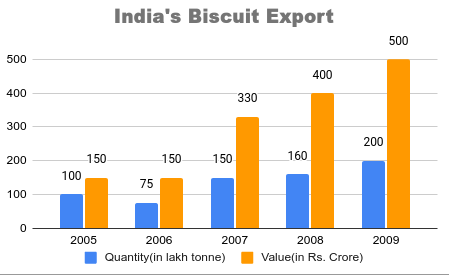What was the percentage drop in the export quantity from 2005 to 2006?

a) 75%

b) 100%

c) 50%

d) 25%

Solution:

From the bar graph,

Export quantity of India in 2005 = 100

Export quantity of India in 2006 = 75

$\therefore\$Required percentage drop = $\frac{100-75}{100}\times100=$ 25%

Hence, the correct answer is Option D

Question 12: The given bar graph shows the biscuit exports of India over a period of five years. Study the graph and answer the question that follow.In which year, the percentage increase in exports was maximum from its preceding year:

a) 2007

b) 2006

c) 2009

d) 2008

Solution:

From the bar graph,

Percentage increase in exports from 2006 to 2007 = $\frac{150-75}{75}\times100=$ 100%

Percentage increase in exports from 2007 to 2008 = $\frac{160-150}{150}\times100=$ 6.66%

Percentage increase in exports from 2008 to 2009 = $\frac{200-160}{200}\times100=$ 20%

From 2005 to 2006, there was decrease in exports.

$\therefore\$The percentage increase in exports was maximum in 2007 from its preceding year.

Hence, the correct answer is Option A

Question 13: The given bar-graph represents the number of teachers in different weight groups. Study the graph and answer the question that follows.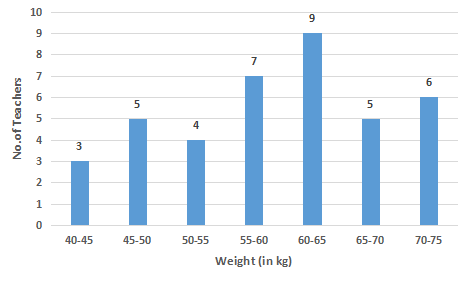In which of the following weight groups is the number of teachers the maximum?

a) 45 – 50

b) 60 – 65

c) 65 – 70

d) 40 – 45

Solution:

From the bar graph,

Number of teachers in the weight group 45 – 50 = 5

Number of teachers in the weight group 60 – 65 = 9

Number of teachers in the weight group 65 – 70 = 5

Number of teachers in the weight group 40 – 45 = 3

$\therefore\$The number of teachers in the weight group 60 – 65 is highest.

Hence, the correct answer is Option B

Question 14: The given bar graph shows the biscuit exports of India over a period of five years. Study the graph and answer the question that follow.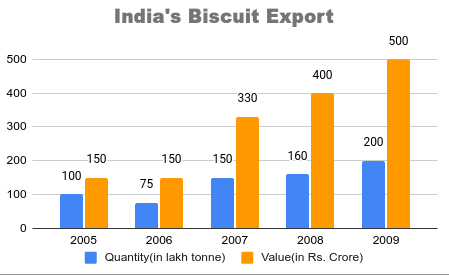In which two years was the value per tonne equal?

a) 2006 and 2007

b) 2008 and 2009

c) 2007 and 2008

d) 2005 and 2006

Solution:

From the bar graph,

Value per tonne of the exports in 2005 = $\frac{150}{100}=1.5$ crore/lakh tonne = 1.5 x 10$^{-5}$ crore/tonne

Value per tonne of the exports in 2006 = $\frac{150}{75}=2$ crore/lakh tonne = 2 x 10$^{-5}$ crore/tonne

Value per tonne of the exports in 2007 = $\frac{330}{150}=2.2$ crore/lakh tonne = 2.2 x 10$^{-5}$ crore/tonne

Value per tonne of the exports in 2008 = $\frac{400}{160}=2.5$ crore/lakh tonne = 2.5 x 10$^{-5}$ crore/tonne

Value per tonne of the exports in 2009 = $\frac{500}{200}=2.5$ crore/lakh tonne = 2.5 x 10$^{-5}$ crore/tonne

$\therefore\$In 2008 and 2009, value per tonne is equal.

Hence, the correct answer is Option B

Question 15: Study the given bar chart and answer the question that follows.
Production of Fertilizers by a Company (in 10,000 tonnes) Over the Years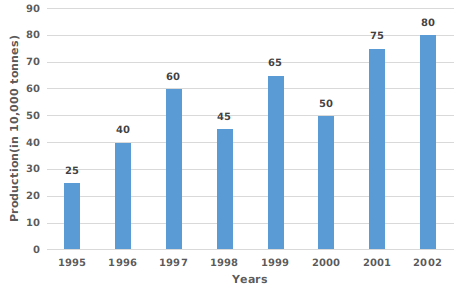What was the approximate percentage increase in the production of fertilizers from 1998 to 1999?

a) 44.4%

b) 50%

c) 40%

d) 48.4%

Solution:

From the bar chart,

Production of fertilizers by the company(in 10,000 tonnes) in 1998 = 45

Production of fertilizers by the company(in 10,000 tonnes) in 1999 = 65

Increase in the production of fertilizers of the company from 1998 to 1999 = 65 – 45 = 20

$\therefore\$Required percentage $=\frac{20}{45}\times100=$ 44.44%

Hence, the correct answer is Option A